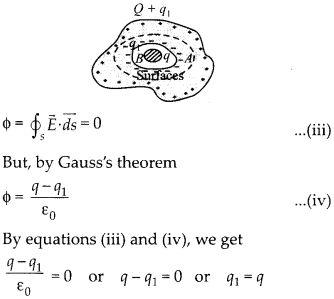Enlightened

# Question 28: NCERT Class 12th Physics: Chapter 1 – Electric Charges And Fields

• 0

Question 28: NCERT Class 12th Physics: Chapter 1 – Electric Charges And Fields

(a) A conductor A with a cavity is shown in figure (a) is given a charge Q. Show that the entire charge must appear on the outer surface of the conductor.
(b) Another conductor B with charge q is inserted into the cavity keeping B insulated from A. Show that the total charge on the outside surface of A is Q + q. (figure (b))
(c) A sensitive instrument is to be shielded from the strong electrostatic fields in its environment. Suggest a possible way.

Share

1. Solution:
(a) Let us consider a closed surface inside the conductor enclosing the cavity. As electric field inside the conductor is zero,This shows that there cannot be any charge on the inner surface of conductor at the cavity, so any charge Q given to the conductor will appear only on its outer surface.
(b) Let us consider that the charge -qI is induced on the inner surface of conductor A at the cavity, when conductor B of charge q is kept in its cavity. Due to this charge of Q + qt is induced on the outer surface of conductor. Let us construct a closed Gaussian surface S inside the conductor A enclosing its cavity. As, electric field inside conductor is zero, soi.e., equal and opposite charge, -q is induced on inner surface of conductor A at the cavity,

when conductor B of charge, + q is kept is its cavity. Hence, the charge on outer surface of conductor is Q + q1 = Q + q
(c) As we found that electric field inside the cavity of conductor is zero, even on charging the conductor, so the sensitive instrument can be shielded from the strong electrostatic fields in its environment, by covering it with a metallic cover.

Check the complete chapter with solutions.

NCERT Solutions for 12th Class Physics: Chapter 1-Electric Charges And Fields

• 0